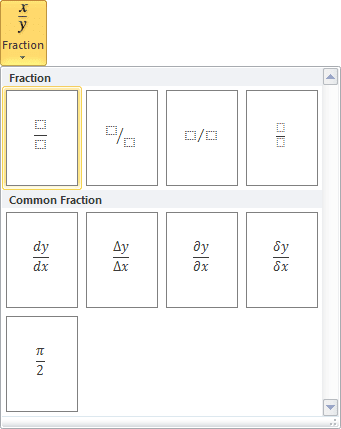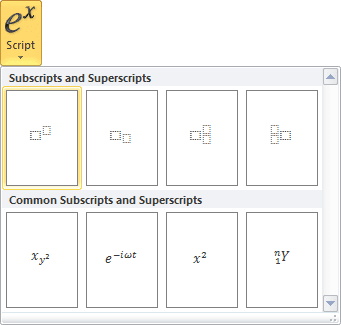# How to insert an equation with fractions, square roots and exponents

Word
This tip display how to insert an equation for example, the normal, or Gaussian distribution.

How to add an equation in your document, see Working with Microsoft Equation.

To insert, for example, the normal, or Gaussian distribution, do the following:

In the Professional present:

1.   In your own equation enter f(x)=.

2.   On the Equation Tools Design tab, in the Structures group, click the Fraction button:In the Fraction list choose Stacked Fraction:3.   Enter 1 in the top of your fraction.

4.   In the bottom of your fraction, do the following:

4.1.   On the Equation Tools Design tab, in the Structures group, click the Radical button. In the Radical list choose Square root:4.2.   Enter 2.

4.3.   On the Equation Tools Design tab, in the Symbols group, click the More button:In the list of symbols choose:4.4.   On the Equation Tools Design tab, in the Structures group, click the Script button. In the Script list choose Superscript:4.5.   In the base box of script choose.

4.6.   In the upper right box of script enter 2.

5.   In the left of your formula choose Script again to enter e in the base box, in the upper right box enter - and choose again Fraction etc.:In the Linear present:

1.   In your own equation enter f(x)=1/.

2.   On the Equation Tools Design tab, in the Symbols group, chooseor simply \sqrt.

3.   In the brackets enter 2(or \pi),(or \sigma) and ^2:Then you enter a space key, this linear formula transformed to the professional format:4.   Enter e^(-(x-(or \mu), )^2/(2,(or \sigma) and then ^2)):Then you enter a space key, second part of your linear formula transformed to the professional format:If you have any questions or suggestions, please feel free to ask OfficeToolTips team.